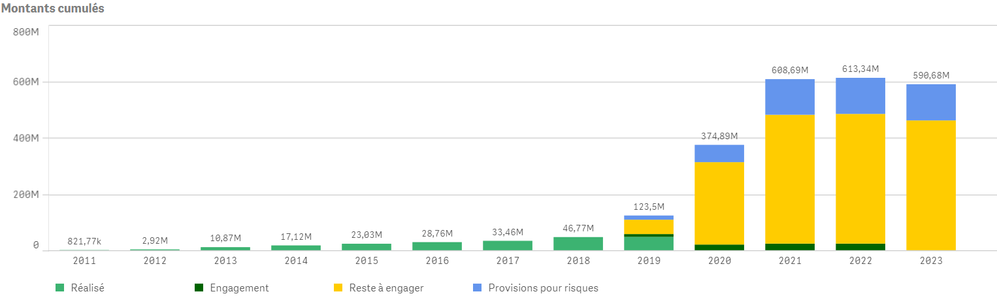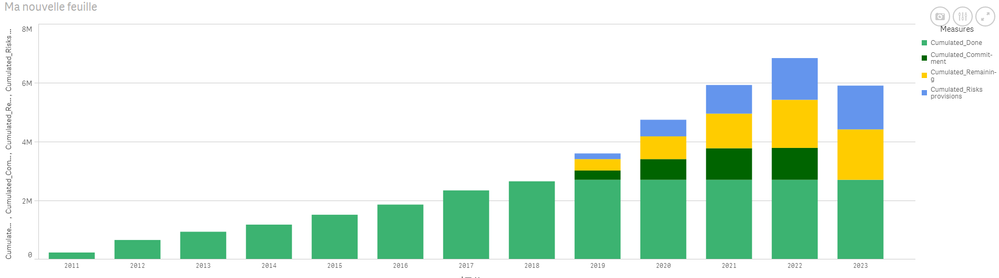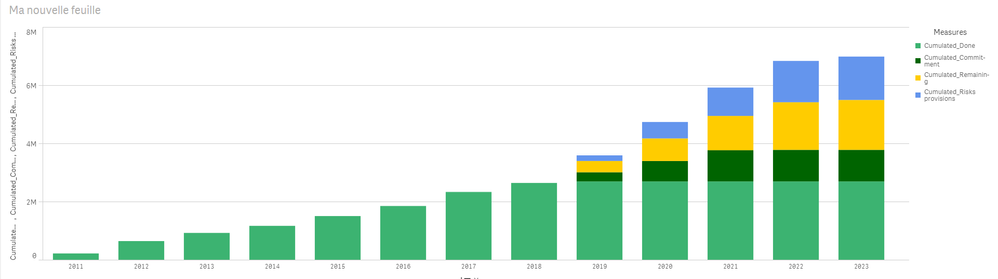# New to Qlik Sense

If you’re new to Qlik Sense, start with this Discussion Board and get up-to-speed quickly.

Announcements
Skip the ticket, Chat with Qlik Support instead for instant assistance.
cancel
Showing results for
Did you mean:Partner - Contributor III

## Cumulative sum with several measures

Hi everyone,

I'm learing how to use QlikSense, and have used a lot of what I've found here : thank you to everyone in this community.

I'm currently facing an issue and can't seem to find a solution to my problem.

Context :

My main measure is named "Amount". It roughly represents budget.

The amount is linked to a date in which the amount is supposed to be spent.
There are several categories to the amount : Spent, Planned, To be planned, Planned for risks.

What I need to do :

I am trying to make a bar graph with cumulated values of the amount, split by each category.

What I've tried :
I have been using the following formula for each type of amount :

Sum(Aggr( Rangesum(Above(Sum({\$<Year=,"Amount_type"={'Spent'}>}Amount) ,0,\$(=Count(Distinct {1} Year)))), (Year, (Numeric, Ascending))))

See belowMy issue :

As you can see, is that the light green (Réalisé = 'spent') for example does not carry in 2020 and above.
While I don't have any 'spent' amount in 2020 and above, I don't understand why it does not keep the 2019 value, and what I need to do to keep it.

Alexis

Labels (2)

• ### Set Analysis

1 Solution

Accepted SolutionsMVP

Isn't this the goal?I changed the expression for 'Cumulated_Done' to this

```If(GetSelectedCount([Year])=1,
Sum(Aggr(RangeSum(Above(Sum({\$<YearMonth, [Amount Type] = {'Done'}>} [Amount]), 0, \$(=Count(Distinct {1} Year)*12))) + Sum(0), (YearMonth, (LOAD_ORDER, Ascending)))),
Sum(Aggr(RangeSum(Above(Sum({\$<Year, [Amount Type] = {'Done'}>} [Amount]), 0, \$(=Count(Distinct {1} Year)))) + Sum(0), (Year, (Numeric, Ascending))))
)```
9 RepliesMVP

Can you try this and see if this works

```Sum(Aggr(
Rangesum(Above(
Sum({\$<Year, "Amount_type" = {'Spent'}>} Amount) + Sum(0)
, 0, \$(=Count(Distinct {1} Year))))
, (Year, (Numeric, Ascending))))```Partner - Contributor III
Author

Hello,

I have tried, but it has had no effect on my graph.
What was it supposed to make ?MVP

I hoped it would start showing the missing bar for Réalisé. This could be an issue related to the missing data, but difficult to say without having to look. Would you be able to share a sample?Partner - Contributor III
Author

Hello,

Here you can find somme dumy data :

• Date file
• Amount file
• According qvf

I have created several master elements :

• master dimension date : Year / YearMonth
• Master measures :
• my 4 sum amounts with Amount types
• my 4 cumulated values (there is an if statement to keep a cumulated value on the year if I have only one selected)

I hope this helps

Thanks a lot for your helpMVP

Isn't this the goal?I changed the expression for 'Cumulated_Done' to this

```If(GetSelectedCount([Year])=1,
Sum(Aggr(RangeSum(Above(Sum({\$<YearMonth, [Amount Type] = {'Done'}>} [Amount]), 0, \$(=Count(Distinct {1} Year)*12))) + Sum(0), (YearMonth, (LOAD_ORDER, Ascending)))),
Sum(Aggr(RangeSum(Above(Sum({\$<Year, [Amount Type] = {'Done'}>} [Amount]), 0, \$(=Count(Distinct {1} Year)))) + Sum(0), (Year, (Numeric, Ascending))))
)```MVP

Updated all the expression because I saw that even 'Cumulated_Commitment' was missing for 2023Partner - Contributor III
Author

Amazing, thank you very much !

Could you please share how it works ? I'm not sure to understandMVP

Since Amount Type = 'Done' was non existent for the years 2020 onwards, the expression was treated as null and null cannot be accumulated with a number. Since we knew that there are other Amount Types which are not null for the other period, I added Sum(0) which will change the expression from null to 0. Now 0 can be accumulated with other numbers.

Does that make sense?Partner - Contributor III
Author

Yes that makes perfect sense, thank you !

I find that it is a very non-intuitive way of implementing it, but that is another story 🙂Tags
Community Browser